# Ncert Class 6 Math Understanding Elementary Shapes Exercise 5.4

Understanding Elementary Shapes Class 6 Ex. 5.4

New Ncert Class 6 Math Free Solution.

Exercise 5.4

Question 1 :- What is the measure of :
(i) a right angle
(ii) a straight angle?

Solution 1:-

(i)  Measure of a right angle = 90°
(ii) Measure of a straight angle is 180 degree.

Question 2 :- Say True or False:
(a) The measure of an acute angle < 90°
(b) The measure of an obtuse angle < 90°
(c) The measure of a reflex angle > 180°
(d) The measure of one complete revolution = 360°
(e) If m ∠A = 53° and m ∠B = 35°, then m ∠A > m ∠B.

Solution 2:-

(a) True
(b) False
(c) True
(d) True
(e) True

Question 3 :- Write down the measures of
(a) some acute angles
(b) some obtuse angles
(give at least two examples of each).

Solution 3:-

(a) 23°, 89° are acute angles.
(b) 91°, 179° are obtuse angles.

Question 4 :- Measure the angles given below using the protractor and write down the measure.

(a)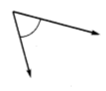(b)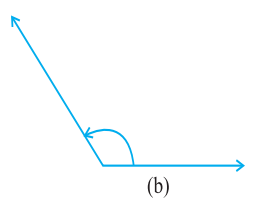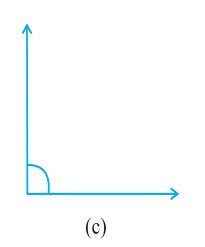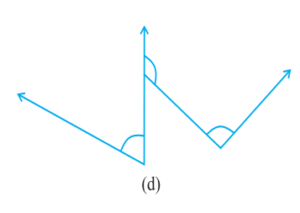Solution 4:-
(a) 45°
(b) 125°
(c) 90°
(d) ∠1 = 60°, ∠2 = 90°, ∠3 = 125°

Question 5 :- Which angle has a large measure? First estimate and then measure.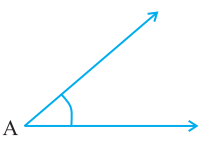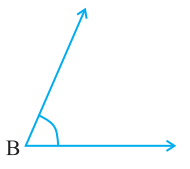Measure of Angle A =
Measure of Angle B =

Solution 5:-

Measure of Angle A = 40°
Measure of Angle B = 60°.

Question 6 :- From these two angles which has larger measure? Estimate and then confirm by measuring them.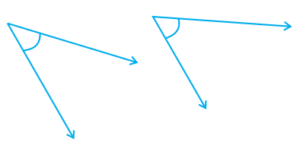(a)                              (b)
Solution 6:-
Angle (b)’s opening is larger than that of angle (a).

Measure of angle (a) = 45°
Measure of angle (b) = 60°

Question 7 :- Fill in the blanks with acute, obtuse, right or straight:
(a) An angle whose measure is less than that of a right angle is …………….. .
(b) An angle whose measure is greater than that of a right angle is ……….. .
(c) An angle whose measure is the sum of the measures of two right angles is …………….. .
(d) When the sum of the measures of two angles is that of a right angle, then each one of them is ……. .
(e) When the sum of the measures of two angles is that of a straight angle and if one of them is acute then the other should be …………….. .

Solution 7:-

(a) acute
(b) obtuse (if the angle is less than 180 degree)
(c) straight
(d) acute
(e) obtuse

Question 8 :- Find the measure of the angle shown in each figure. (First estimate with your eyes and than find the actual measure with a protractor).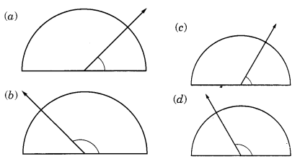Solution 8:-
(a) Measure of the angle = 40°
(b) Measure of the angle = 130°
(c) Measure of the angle = 65°
(d) Measure of the angle = 135°.

Question 9 :- Find the angle measure between the hands of the clock in each figure: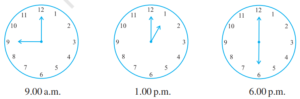Solution 9:-
1.) At 9:00 a.m., a clock’s hour hand and minute hand make a 90° angle.
2.) At 1:00 p.m., a clock’s hour hand and minute hand make a 30° angle.
3.) At 6:00 p.m., a clock’s hour hand and minute hand make a 180° angle.

Question 10 :- Investigate:
In the given figure, the angle measures 30°. Look at the same figure through a magnifying glass. Does the angle becomes larger? Does the size of the angle change?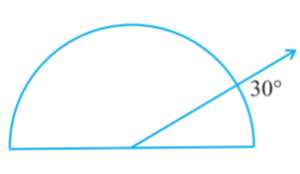Solution 10:-

The angle measurement will remain unchanged when seen with a magnifying glass.

Question 11:- Measure and classify each angle: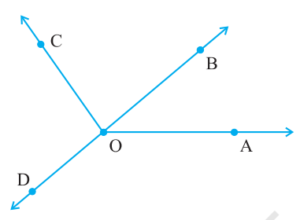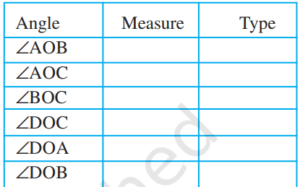Solution 11:-

1. ∠AOB = 40 degree (acute angle).
2. ∠AOC = 125 degree (obtuse angle)
3. ∠BOC = 85 degree (acute angle)
4. ∠DOC = 95 degree (obtuse angle)
5. ∠DOA = 140 degree (obtuse angle)
6. ∠DOB = 180 degree (straight angle)

Free Free Free